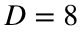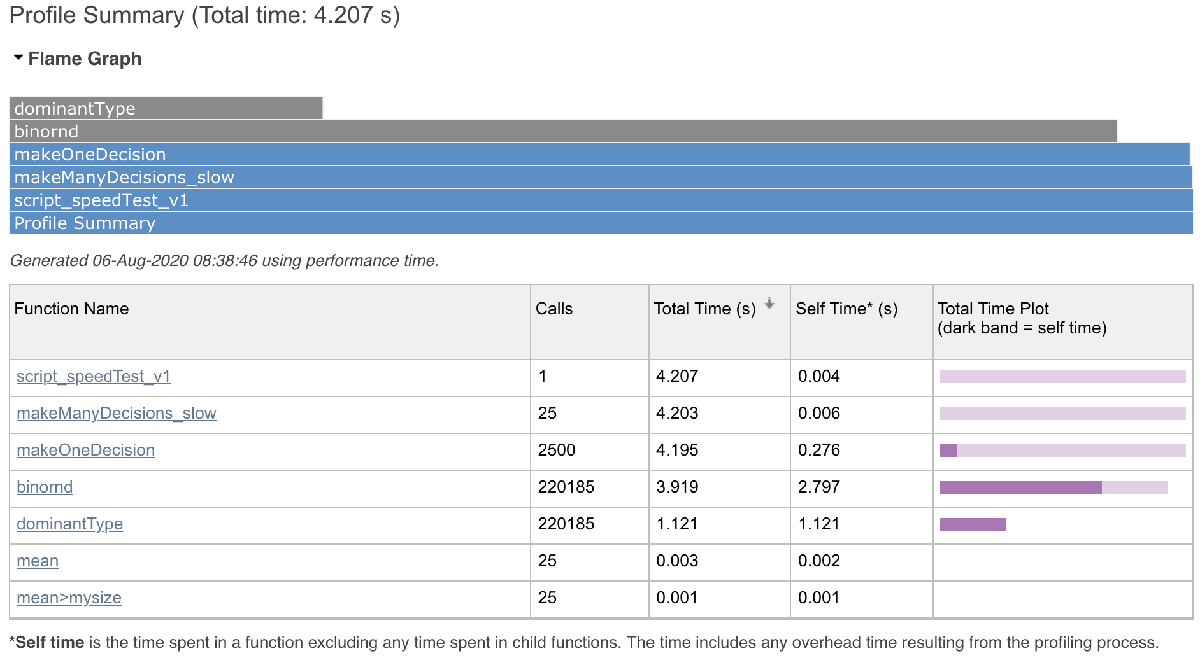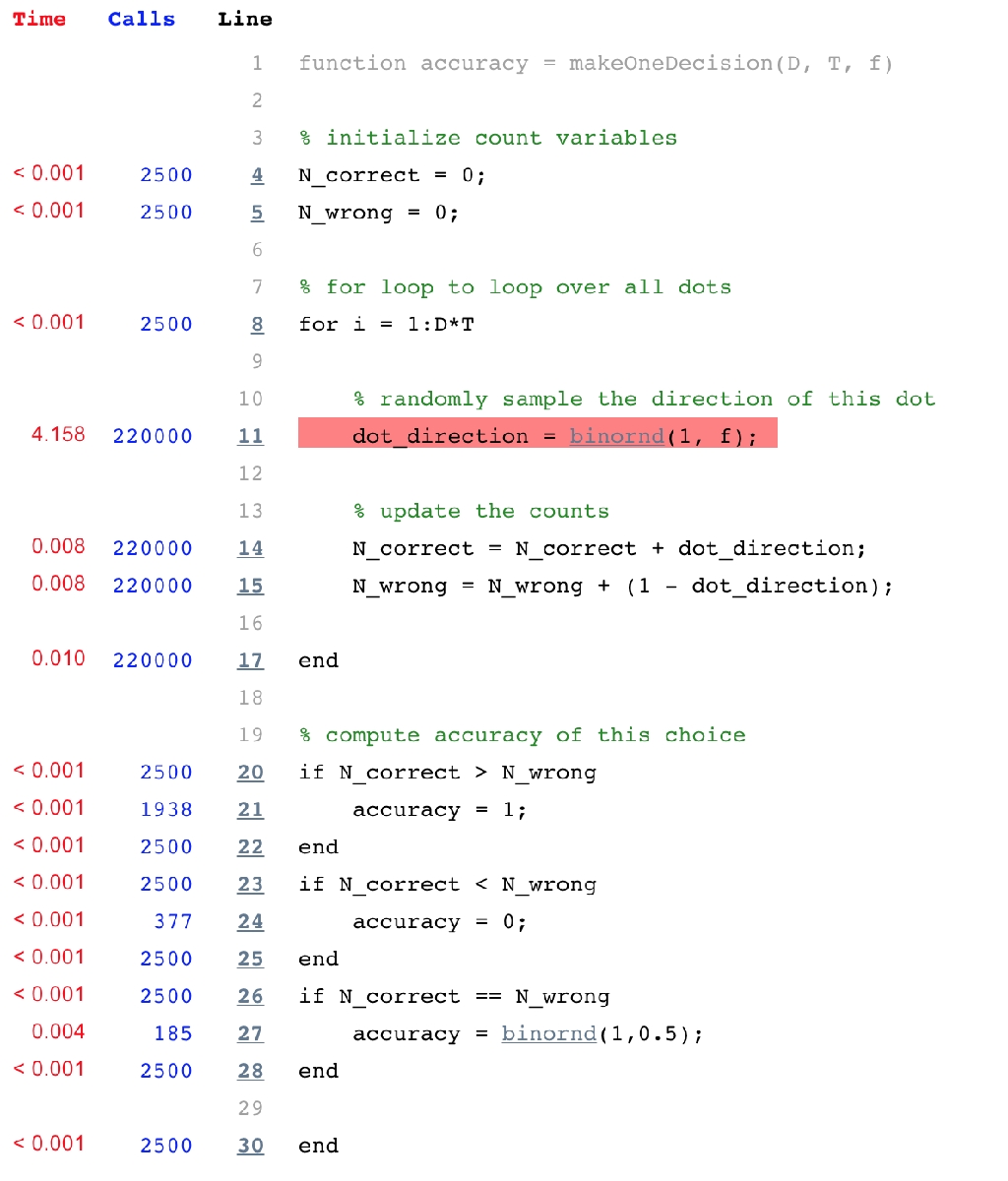# Assignment 2: Modeling a full experiment

*** Due date: Start of class in Week 3 ***

## Part 1: Create a function for one decision (2 points)

• Create a new directory for this week's work. Call it Week_02
• Follow along with the class material to make a function to implement one decision (call it makeOneDecision) and make sure you give it inputs for D, T, and f.
• Make a script to test your makeOneDecision function. This script should print the accuracy of the one decision to the Command Window.
Note you can output the accuracy by just typing a single line:
accuracy
without the semi colon at the end.

## Part 2: Create a function to make many decisions (3 points)

• Make a new function to make many decisions (call it makeManyDecisions).
• Use a for loop around your makeOneDecision function, something like this perhaps
for i = 1:nDecisions
accuracy(i) = makeOneDecision(D, T, f);
end
• Then create a new variable for the mean of the accuracy across all of your trials and set it as the output of the function
• Make a script to test your makeManyDecisions function.

## Part 3: Create a script to simulate behavior for many different values of T and f (3 points)

• Follow along with the material to make a script that computes average accuracy for many different values of T and f.
• Usefor the number of dots, and nDecisions = 100
Note: Depending on how fast your computer is, your code may take a while to run. If it's taking a very long time (more than 5 minutes) you can speed things up by reducing nDecisions to 50 or less.
Note: If you want to stop the code running before it's done, click on the Command Window and press control+C (on mac and on windows).
• Plot the average accuracy as a function of T and f and use the commands in the make the plot look "nice"

## Part 4: Compare the behavior of your model with the behavior of the monkeys from Gold and Shadlen (2 points)

• Now that your model has performed the experiment, we can compare it's behavior with the behavior of the monkeys from Gold and Shadlen. To do this we need the actual data from that paper. To get this, download the file
• from D2L and save it in your Week_02 directory.
• In your script, after the code to make your plot, write
• This will load the data points from the plot. This data has two variables, dataX for the location of each point on the x axis and dataY for the location on the y axis.
• Now plot the data on top of your simulated data. To do this you'll need to use the command
hold on
• hold on stops the figure from being cleared when you add something new to the plot
• After the hold on, plot the data on the same axes as your simulated data using
l2 = plot(dataX, dataY, '.');
• The dot '.' will make the plot a bunch of dots rather than lines.
• Change the colors of the Shadlen and Gold data points to match the corresponding lines for your model; e.g.
set(l2(1), 'color', AZ_chili)
• You can also change the size of the markers using
set(l2, 'markersize', 30)
• Finally, take a look at your plot. How well does your simulated data agree with the monkey data? In a comment in your code say whether you think this fit is good or not and try to think of a way in which you could improve the fit.

## Part 5: Speed up your code and improve the model (2 extra credit bonus points)

Note: This extra credit item is more for expert coders, feel free to try it if you are a novice, but you might need to reach out the instructors for help.
You may have noticed that the code we wrote this week runs pretty slow. The reason for this is that Matlab does pretty badly with for loops. However, there are ways to speed things up (by up to a factor of 500 times!) by replacing the for loops with vectors and matrices.
Let's start with our makeOneDecision function. First of all let's find out what lines are slowing us down by timing our code. To do this, go to the EDITOR tab on the toolbar and hit "Run and Time."
After it's run (which takes slightly longer than to run your code without timing) you'll get something like this pop up ...This is the code profiler that let's you see which lines of code are taking the longest ... if you click on the link for "makeOneDecision" and scroll down, you'll see something like this ...This is a line-by-line breakdown of the makeOneDecision function with the time taken to run each line. From this you can clearly see that it's the binornd line that's taking so long - 4.158 seconds on my machine, or about 99% of the entire running time!
The reason this line takes so long is because we are calling it so many times (220,000 times). It's likely that we can speed things up considerably if we can avoid calling binornd quite so many times ...
To do this we need to use a vector version of binornd, to generate all the random numbers we need in one go, which turns out to be way quicker than generating one random number at a time. We can do this like this ...
dot_direction = binornd(1, f, T*D, 1);
This will generate a vector of dot directions, one for each dot. We can then compute N_correct as
N_correct = sum(dot_direction);
and N_wrong as
N_wrong = sum( 1 - dot_direction );
Note: the sum function just sums up all the elements of a vector.
Have a play around with this and see how much it speeds up your code.
Then, if you are feeling brave, have a go at speeding up makeManyDecisions by bypassing makeOneDecision entirely and generating a matrix of dot directions, one for each dot on each trial using
dot_direction = binornd(1, f, T*D, nDecisions);
You'll then need to use the sum function to sum over the first dimension of dot_direction to get a vector of N_corrects and N_wrong.
N_correct = sum(dot_direction);
If you manage to speed up makeManyDecisions, try running your code with a much bigger number for nDecisions, (1000 or even 10,000 is good) and more T_vals so you can really map out each line. This will really let you see where the model fails to capture the data.
Finally, see if you can get the model fit to be even better by including one more variable - a dead time in which the monkey ignores the input stimulus. This means that for a given viewing time, the actual time the monkey sees the stimulus is reduced by a constant amount. See if you can include this dead time into your simulations. If you can, you'll find you can fit the data almost perfectly!